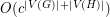# algorithm

## Algorithm for graph homomorphisms ★★

Author(s): Fomin; Heggernes; Kratsch

Question

Is there an algorithm that decides, for input graphsand, whether there exists a homomorphism fromtoin timefor some constant?

## Is there an algorithm to determine if a triangulated 4-manifold is combinatorially equivalent to the 4-sphere? ★★★

Author(s): Novikov

Problem   Is there an algorithm which takes as input a triangulated 4-manifold, and determines whether or not this manifold is combinatorially equivalent to the 4-sphere?

Keywords: 4-sphere; algorithm